##帐号 自动登录 找回密码 密码 立即注册→加入我们
 搜索 热搜: 下载 VB C 实现 编写

# 【翻译】Z3求解器

1主题 133积分

UID
2054

0

2 点

102 个

0 次

0 份

15 小时

2016-11-10发表于 2018-3-12 18:52:29 | 显示全部楼层 |阅读模式

### 欢迎访问技术宅的结界，请注册或者登录吧。

x

Z3完整的API文档在这：http://z3prover.github.io/api/html/index.html

# Z3 API in Python

Z3是微软研究院开发的一个高效的定理证明器。Z3可以被用于很多场景，例如：软件/硬件测试、约束求解、混合系统分析、安全、生物（药物设计）和几何问题。

Z3同样包含了C、.net和OCaml的API。Z3Py的源代码可以在Z3发行版中看到，你可以按照你的需求进行修改。源代码也展示了如何使用Z3 4.0的新功能。其他酷炫的Z3前端有ScalaZ3和SBV。

###入门

python
x = Int('x')
y = Int('y')
solve(x > 2, y < 10, x + 2*y == 7)


python
x = Int('x')
y = Int('y')
print simplify(x + y + 2*x + 3)
print simplify(x < y + x + 2)
print simplify(And(x + 1 >= 3, x**2 + x**2 + y**2 + 2 >= 5))


python
set_option(html_mode=False)


python
x = Int('x')
y = Int('y')
print x**2 + y**2 >= 1
set_option(html_mode=False)
print x**2 + y**2 >= 1py


Z3提供拆分表达式的函数

python
x = Int('x')
y = Int('y')
n = x + y >= 3
print "num args: ", n.num_args()
print "children: ", n.children()
print "1st child:", n.arg(0)
print "2nd child:", n.arg(1)
print "operator: ", n.decl()
print "op name:  ", n.decl().name()


Z3支持所有基础数学运算。Z3Py使用与Python相同的运算优先级。如Python中，**是乘幂。Z3可以求解非线性多项式约束。

python
x = Real('x')
y = Real('y')
solve(x**2 + y**2 > 3, x**3 + y < 5)


**Real('x')** 建立一个实变量x。Z3Py可以表示任意大的整数，有理数（如上面例子所示），代数数。代数数是指任何整系数多项式的复根。Z3内部精确的表示了每个数。无理数被显示为十进制使我们较容易读出结果。

python
x = Real('x')
y = Real('y')
solve(x**2 + y**2 == 3, x**3 == 2)

set_option(precision=30)
print "Solving, and displaying result with 30 decimal places"
solve(x**2 + y**2 == 3, x**3 == 2)


**set_option** 指令用于设置Z3环境。它被用于设置全局属性，如结果如何显示等。指令

python
set_option(precision=30)


python
print 1/3
print RealVal(1)/3
print Q(1,3)

x = Real('x')
print x + 1/3
print x + Q(1,3)
print x + "1/3"
print x + 0.25


python
x = Real('x')
solve(3*x == 1)

set_option(rational_to_decimal=True)
solve(3*x == 1)

set_option(precision=30)
solve(3*x == 1)


python
x = Real('x')
solve(x > 4, x < 0)


python
# This is a comment
x = Real('x') # comment: creating x
print x**2 + 2*x + 2  # comment: printing polynomial


### 逻辑运算

Z3支持的逻辑运算符：And,Or,Not,Implies(蕴含),If(if-then-else)。双蕴含用 **==** 表示。下面的例子展示了如何求解一个布尔约束。

python
p = Bool('p')
q = Bool('q')
r = Bool('r')
solve(Implies(p, q), r == Not(q), Or(Not(p), r))


Python中的布尔常量 **True** 和 **False** 可以用于Z3表达式。

python
p = Bool('p')
q = Bool('q')
print And(p, q, True)
print simplify(And(p, q, True))
print simplify(And(p, False))


python
p = Bool('p')
x = Real('x')
solve(Or(x < 5, x > 10), Or(p, x**2 == 2), Not(p))


### 求解器

Z3提供了不同的求解器。上述例子中使用的命令 **solve** 调用了Z3求解器的API。这些调用可以在Z3目录下的 **z3.py** 中找到。

python
x = Int('x')
y = Int('y')

s = Solver()
print s

s.add(x > 10, y == x + 2)
print s
print "Solving constraints in the solver s ..."
print s.check()

print "Create a new scope..."
s.push()
s.add(y < 11)
print s
print "Solving updated set of constraints..."
print s.check()

print "Restoring state..."
s.pop()
print s
print "Solving restored set of constraints..."
print s.check()


**Solver()** 命令定义了一个通用的求解器。所有约束式可以通过 **add** 方法添加。我们称约束式在求解器中 **被声明**。**check()** 方法求解所有已添加进求解器的约束式。如果可以求得解，该方法返回 **sat** 。如果不能求出解则返回 **unsat** 。也有可能出现声明的约束式不成立的情况，这时求解器返回 **unknown** 。

python
x = Real('x')
s = Solver()
s.add(2**x == 3)
print s.check()


python
x = Real('x')
y = Real('y')
s = Solver()
s.add(x > 1, y > 1, Or(x + y > 3, x - y < 2))
print "asserted constraints..."
for c in s.assertions():
print c

print s.check()
print "statistics for the last check method..."
print s.statistics()
# Traversing statistics
for k, v in s.statistics():
print "%s : %s" % (k, v)


python
x, y, z = Reals('x y z')
s = Solver()
s.add(x > 1, y > 1, x + y > 3, z - x < 10)
print s.check()

m = s.model()
print "x = %s" % m[x]

print "traversing model..."
for d in m.decls():
print "%s = %s" % (d.name(), m[d])


python
x = Real('x')
y = Real('y')
z = Real('z')


### 计算

Z3支持实型和整型变量。在单个问题中两种变量可以混合使用。与大多数编程语言一样，当我们需要时，Z3Py会自动地将整型表达式强制转换为实型。下面的例子展示了各种声明整型和实型变量的方法。

python
x = Real('x')
y = Int('y')
a, b, c = Reals('a b c')
s, r = Ints('s r')
print x + y + 1 + (a + s)
print ToReal(y) + c


Z3Py支持所有的基本的运算符。

python
a, b, c = Ints('a b c')
d, e = Reals('d e')
solve(a > b + 2,
a == 2*c + 10,
c + b <= 1000,
d >= e)


python
x, y = Reals('x y')
# Put expression in sum-of-monomials form
t = simplify((x + y)**3, som=True)
print t
# Use power operator
t = simplify(t, mul_to_power=True)
print t


**help_simplify** 函数打印**simplify** 函数所有可用的参数。Z3Py允许用户以两种方式写入这些参数。Z3内部的参数名以**:** 开头并以**-** 隔开各个单词。这些参数可以被Z3Py使用。Z3Py也提供了一些符合Python变量命名规则的名字，在这里**:** 被移除，**-** 被**_** 取代。下面的例子展示了怎样使用以这两种方式调用函数。

python
x, y = Reals('x y')
# Using Z3 native option names
print simplify(x == y + 2, ':arith-lhs', True)
# Using Z3Py option names
print simplify(x == y + 2, arith_lhs=True)

print "\nAll available options:"
help_simplify()


Z3Py可以支持任意大的数字。下面的例子展示了使用大数，有理数和无理数进行基本运算。无理数中，Z3Py只支持代数数。代数数已经足够表示多项式约束的解了。Z3Py总是以十进制显示无理数，因为这方便阅读。可以使用 **sexpr()** 方法提取Z3内部的表达式，它能以S-表达式（s-expression）的形式显示Z3内部的数学表达式。

python
x, y = Reals('x y')
solve(x + 10000000000000000000000 == y, y > 20000000000000000)

print Sqrt(2) + Sqrt(3)
print simplify(Sqrt(2) + Sqrt(3))
print simplify(Sqrt(2) + Sqrt(3)).sexpr()
# The sexpr() method is available for any Z3 expression
print (x + Sqrt(y) * 2).sexpr()


### 硬件相关的运算

（注：此处为意译）

python
x = BitVec('x', 16)
y = BitVec('y', 16)
print x + 2
# Internal representation
print (x + 2).sexpr()

# -1 is equal to 65535 for 16-bit integers
print simplify(x + y - 1)

# Creating bit-vector constants
a = BitVecVal(-1, 16)
b = BitVecVal(65535, 16)
print simplify(a == b)

a = BitVecVal(-1, 32)
b = BitVecVal(65535, 32)
# -1 is not equal to 65535 for 32-bit integers
print simplify(a == b)


python
# Create to bit-vectors of size 32
x, y = BitVecs('x y', 32)

solve(x + y == 2, x > 0, y > 0)

# Bit-wise operators
# & bit-wise and
# | bit-wise or
# ~ bit-wise not
solve(x & y == ~y)

solve(x < 0)

# using unsigned version of <
solve(ULT(x, 0))


python
# Create to bit-vectors of size 32
x, y = BitVecs('x y', 32)

solve(x >> 2 == 3)

solve(x << 2 == 3)

solve(x << 2 == 24)


### 函数

python
x = Int('x')
y = Int('y')
f = Function('f', IntSort(), IntSort())
solve(f(f(x)) == x, f(x) == y, x != y)


（注：这段因为涉及到一阶逻辑的知识翻了好久还是一头雾水，不过看了下代码，这段大概的意思应该就是，Z3的输入可以是一个未定义具体内容的函数，它可以通过与该函数相关的一些约束式来求出符合要求的函数模型）

python
x = Int('x')
y = Int('y')
f = Function('f', IntSort(), IntSort())
s = Solver()
s.add(f(f(x)) == x, f(x) == y, x != y)
print s.check()
m = s.model()
print "f(f(x)) =", m.evaluate(f(f(x)))
print "f(x)    =", m.evaluate(f(x))


###可满足性和有效性

python
p, q = Bools('p q')
demorgan = And(p, q) == Not(Or(Not(p), Not(q)))
print demorgan

def prove(f):
s = Solver()
s.add(Not(f))
if s.check() == unsat:
print "proved"
else:
print "failed to prove"

print "Proving demorgan..."
prove(demorgan)


### 列表解析式

Python支持列表解析式。列表解析式为创建列表提供了一个简便的方法。它们可以用于创建Z3表达式。下面的例子展示了如何在Z3Py中使用过列表解析式。

python
Create list [1, ..., 5]
print [ x + 1 for x in range(5) ]

# Create two lists containg 5 integer variables
X = [ Int('x%s' % i) for i in range(5) ]
Y = [ Int('y%s' % i) for i in range(5) ]
print X

# Create a list containing X[i]+Y[i]
X_plus_Y = [ X[i] + Y[i] for i in range(5) ]
print X_plus_Y

# Create a list containing X[i] > Y[i]
X_gt_Y = [ X[i] > Y[i] for i in range(5) ]
print X_gt_Y

print And(X_gt_Y)

# Create a 3x3 "matrix" (list of lists) of integer variables
X = [ [ Int("x_%s_%s" % (i+1, j+1)) for j in range(3) ]
for i in range(3) ]
pp(X)


Z3Py也提供创建布尔、整型和实型向量的函数，这些函数可以在列表解析式中被执行。

Python
X = IntVector('x', 5)
Y = RealVector('y', 5)
P = BoolVector('p', 5)
print X
print Y
print P
print [ y**2 for y in Y ]
print Sum([ y**2 for y in Y ])


（注：下面内容为Z3在一些领域应用的示例）

###运动方程

python
d == v_i * t + (a*t**2)/2
v_f == v_i + a*t


#### 例题1

Ima Hurryin以30m/s的速度靠近红绿灯。此时灯变黄，Ima踩下刹车。如果Ima的加速度为-8.00m/s^2，请计算车辆在减速过程中的位移。

python
d, a, t, v_i, v_f = Reals('d a t v__i v__f')

equations = [
d == v_i * t + (a*t**2)/2,
v_f == v_i + a*t,
]
print "Kinematic equations:"
print equations

# Given v_i, v_f and a, find d
problem = [
v_i == 30,
v_f == 0,
a   == -8
]
print "Problem:"
print problem

print "Solution:"
solve(equations + problem)


#### 例题2

Ben Rushin在等红绿灯。当灯变绿时，Ben以6.00m/s^2的加速度加速4.10秒。请计算这段时间Ben的位移。

python
d, a, t, v_i, v_f = Reals('d a t v__i v__f')

equations = [
d == v_i * t + (a*t**2)/2,
v_f == v_i + a*t,
]

# Given v_i, t and a, find d
problem = [
v_i == 0,
t   == 4.10,
a   == 6
]

solve(equations + problem)

# Display rationals in decimal notation
set_option(rational_to_decimal=True)

solve(equations + problem)


###位运算技巧

#### 2的幂

python
x      = BitVec('x', 32)
powers = [ 2**i for i in range(32) ]
fast   = And(x != 0, x & (x - 1) == 0)
slow   = Or([ x == p for p in powers ])
print fast
prove(fast == slow)

print "trying to prove buggy version..."
fast   = x & (x - 1) == 0
prove(fast == slow)


####相反数

python
x      = BitVec('x', 32)
y      = BitVec('y', 32)

# Claim: (x ^ y) < 0 iff x and y have opposite signs
trick  = (x ^ y) < 0

# Naive way to check if x and y have opposite signs
opposite = Or(And(x < 0, y >= 0),
And(x >= 0, y < 0))

prove(trick == opposite)


### 数学问题

#### 猫狗和老鼠

python
# Create 3 integer variables
dog, cat, mouse = Ints('dog cat mouse')
solve(dog >= 1,   # at least one dog
cat >= 1,   # at least one cat
mouse >= 1, # at least one mouse
# we want to buy 100 animals
dog + cat + mouse == 100,
# We have 100 dollars (10000 cents):
#   dogs cost 15 dollars (1500 cents),
#   cats cost 1 dollar (100 cents), and
#   mice cost 25 cents
1500 * dog + 100 * cat + 25 * mouse == 10000)


#### 数独


# 9x9 matrix of integer variables
X = [ [ Int("x_%s_%s" % (i+1, j+1)) for j in range(9) ]
for i in range(9) ]

# each cell contains a value in {1, ..., 9}
cells_c  = [ And(1 <= X[i][j], X[i][j] <= 9)
for i in range(9) for j in range(9) ]

# each row contains a digit at most once
rows_c   = [ Distinct(X[i]) for i in range(9) ]

# each column contains a digit at most once
cols_c   = [ Distinct([ X[i][j] for i in range(9) ])
for j in range(9) ]

# each 3x3 square contains a digit at most once
sq_c     = [ Distinct([ X[3*i0 + i][3*j0 + j]
for i in range(3) for j in range(3) ])
for i0 in range(3) for j0 in range(3) ]

sudoku_c = cells_c + rows_c + cols_c + sq_c

# sudoku instance, we use '0' for empty cells
instance = ((0,0,0,0,9,4,0,3,0),
(0,0,0,5,1,0,0,0,7),
(0,8,9,0,0,0,0,4,0),
(0,0,0,0,0,0,2,0,8),
(0,6,0,2,0,1,0,5,0),
(1,0,2,0,0,0,0,0,0),
(0,7,0,0,0,0,5,2,0),
(9,0,0,0,6,5,0,0,0),
(0,4,0,9,7,0,0,0,0))

instance_c = [ If(instance[i][j] == 0,
True,
X[i][j] == instance[i][j])
for i in range(9) for j in range(9) ]

s = Solver()
s.add(sudoku_c + instance_c)
if s.check() == sat:
m = s.model()
r = [ [ m.evaluate(X[i][j]) for j in range(9) ]
for i in range(9) ]
print_matrix(r)
else:
print "failed to solve"


#### 八皇后问题

python
# We know each queen must be in a different row.
# So, we represent each queen by a single integer: the column position
Q = [ Int('Q_%i' % (i + 1)) for i in range(8) ]

# Each queen is in a column {1, ... 8 }
val_c = [ And(1 <= Q[i], Q[i] <= 8) for i in range(8) ]

# At most one queen per column
col_c = [ Distinct(Q) ]

# Diagonal constraint
diag_c = [ If(i == j,
True,
And(Q[i] - Q[j] != i - j, Q[i] - Q[j] != j - i))
for i in range(8) for j in range(i) ]

solve(val_c + col_c + diag_c)


### 应用：解决安装问题

Z3可以方便地解决安装问题。思路就是给每个包定义一个布尔变量。如果这个包必须被安装，则值为True。如果a包依赖于b，c和z，我们可以写为：

python
DependsOn(a, [b, c, z])


**DependsOn** 用于获取依赖包的条目：

python
def DependsOn(pack, deps):
return And([ Implies(pack, dep) for dep in deps ])


python
And(Implies(a, b), Implies(a, c), Implies(a, z))


python
def Conflict(p1, p2):
return Or(Not(p1), Not(p2))


python
def DependsOn(pack, deps):
return And([ Implies(pack, dep) for dep in deps ])

def Conflict(p1, p2):
return Or(Not(p1), Not(p2))

a, b, c, d, e, f, g, z = Bools('a b c d e f g z')

solve(DependsOn(a, [b, c, z]),
DependsOn(b, [d]),
DependsOn(c, [Or(d, e), Or(f, g)]),
Conflict(d, e),
a, z)


python
DependsOn(c, [Or(d, e), Or(f, g)])


python
def DependsOn(pack, deps):
if is_expr(deps):
return Implies(pack, deps)
else:
return And([ Implies(pack, dep) for dep in deps ])

def Conflict(*packs):
return Or([ Not(pack) for pack in packs ])

a, b, c, d, e, f, g, z = Bools('a b c d e f g z')

def install_check(*problem):
s = Solver()
s.add(*problem)
if s.check() == sat:
m = s.model()
r = []
for x in m:
if is_true(m[x]):
# x is a Z3 declaration
# x() returns the Z3 expression
# x.name() returns a string
r.append(x())
print r
else:
print "invalid installation profile"

print "Check 1"
install_check(DependsOn(a, [b, c, z]),
DependsOn(b, d),
DependsOn(c, [Or(d, e), Or(f, g)]),
Conflict(d, e),
Conflict(d, g),
a, z)

print "Check 2"
install_check(DependsOn(a, [b, c, z]),
DependsOn(b, d),
DependsOn(c, [Or(d, e), Or(f, g)]),
Conflict(d, e),
Conflict(d, g),
a, z, g)


### 在本地使用Z3Py

Z3Py是Z3发行版的一部分。在z3文件夹中**python** 子目录。为了在本地使用，必须在Python脚本中声明

from Z3 import *

z3 python的目录必须在环境变量**PYTHONPATH** 中。Z3Py会自动地搜索Z3运行库（z3.dll（WIN），libz3.so（Linux）或libz3.dylib（OSX））。你也可以使用下述命令手动初始化Z3Py：

python
init("z3.dll")


ref:

ScalaZ3：http://lara.epfl.ch/~psuter/ScalaZ3/

SBV：http://hackage.haskell.org/package/sbv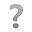25.26 KB, 下载次数: 1

1041主题 5万积分

UID
1

217

288 点

18063 个

36605 次

0 份

1732 小时

2014-1-26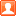发表于 2018-3-12 19:09:32 | 显示全部楼层
 中间因为有[i]这个东西变成了斜体字的BBCode，我看你的帖子似乎也没有使用BBCode进行格式化处理，就把“禁用编辑器代码”勾上了。 然后用查找替换把[url]超链接去掉了，所以链接必须手点。 发代码的时候遇到方括号还是挺尴尬的。

1主题 133积分

UID
2054

0

2 点

102 个

0 次

0 份

15 小时

2016-11-10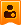楼主| 发表于 2018-3-13 01:15:30 | 显示全部楼层
 0xAA55 发表于 2018-3-12 19:09 中间因为有这个东西变成了斜体字的BBCode，我看你的帖子似乎也没有使用BBCode进行格式化处理，就把“禁用编 ... 比较尴尬= =，因为写的时候是用markdown写的，论坛好像不支持markdown的说

1041主题 5万积分

UID
1

217

288 点

18063 个

36605 次

0 份

1732 小时

2014-1-26发表于 2018-3-13 16:20:42 | 显示全部楼层
 大能猫 发表于 2018-3-13 01:15 比较尴尬= =，因为写的时候是用markdown写的，论坛好像不支持markdown的说 论坛是不支持md的，一般我在这个时候会进行手动的处理。 可以试试用notepad++的录制宏的功能来自动格式化md为BBCode，然后复制粘贴过来就好看了。 但[ i ]斜体字这个是个硬伤，它只能存活于[mw_shl_code]和[code]里面，否则就真的变成斜体字了。 有的帖子我把它换成了［i］来苟且……

1主题 133积分

UID
2054

0

2 点

102 个

0 次

0 份

15 小时

2016-11-10楼主| 发表于 2018-3-14 12:25:13 | 显示全部楼层
 0xAA55 发表于 2018-3-13 16:20 论坛是不支持md的，一般我在这个时候会进行手动的处理。 可以试试用notepad++的录制宏的功能来自动格式化 ... emmm。。。录制宏就是vim里的q么 感觉既然都用录制宏了那不如写个程序处理

1041主题 5万积分

UID
1

217

288 点

18063 个

36605 次

0 份

1732 小时

2014-1-26发表于 2018-3-15 07:53:02 | 显示全部楼层
 大能猫 发表于 2018-3-14 12:25 emmm。。。录制宏就是vim里的q么 感觉既然都用录制宏了那不如写个程序处理 ... 我说的是notepad++的录制宏，关vim鸟事。 这玩意儿是office时代就有的东西，可以记录你的菜单操作、快捷键、查找替换操作等。而且厉害点的还会智能匹配你的请求。论office系列软件（论Windows本身）的正确用法。

1主题 133积分

UID
2054

0

2 点

102 个

0 次

0 份

15 小时

2016-11-10楼主| 发表于 2018-3-16 00:01:45 | 显示全部楼层
 0xAA55 发表于 2018-3-15 07:53 我说的是notepad++的录制宏，关vim鸟事。 这玩意儿是office时代就有的东西，可以记录你的菜单操作、快捷 ... 意思就是功能是不是类似嘛 看来是类似的了

 本版积分规则 回帖并转播 回帖后跳转到最后一页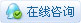|申请友链||Archiver|手机版|小黑屋|技术宅的结界 ( 滇ICP备16008837号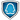|网站地图

GMT+8, 2019-8-21 04:58 , Processed in 0.107086 second(s), 35 queries , Gzip On.

Powered by Discuz! X3.2

© 2001-2013 Comsenz Inc.